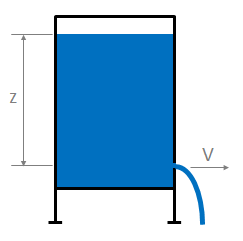# Summary

This article presents Torricelli’s law, a simplified method of estimating the velocity of fluid passing through an open orifice under static pressure.

# Definitions

 : Gravitational acceleration ( ) : Pressure of the fluid ( ) : Velocity of the fluid ( ) : Fluid height ( ) : Density of the fluid ( )

# Torricelli’s Law

Torricelli’s law may be derived from Bernoulli’s principle and relates the velocity of fluid leaving an orifice in a fluid filled container to the height of the fluid above the orifice. This equation is a simplification which will generally hold for simple hole geometries, where pressure losses may be essentially ignored.# Derivation

Torricelli’s Law is derived from the Bernoulli equation by equating the potential energy in the static head of liquid to the kinetic energy of the water leaving through the orifice as follows:

Subsequently rearranging for velocity we have Torricelli’s law:

Article Tags

# Subscribe to our mailing list

 Email Name